Find free online Chemistry Topics covering a broad range of concepts from research institutes around the world.

## What is Stoichiometry? Balancing Equations

Have you ever noticed the preparation of kesari at your home? In one of the popular methods for the preparation of kesari, the required ingredients to prepare six cups of kesari are as follows.

1. Rava – 1 Cup
2. Sugar – 2 Cups
3. Ghee – $$\frac{1}{2}$$ Cup
4. Nuts and Dry Fruits – $$\frac{1}{4}$$ Cup

Otherwise,

1 cup rava + 2 cups sugar + $$\frac{1}{2}$$ cup ghee + $$\frac{1}{4}$$ cup nuts and dry fruits → 6 cups kesari.

From the above information, we will be able to calculate the amount of ingredients that are required for the preparation of 3 cups of kesari as follows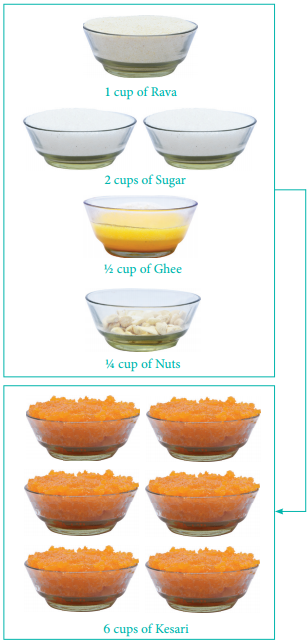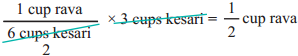Alternatively, we can calculate the amount of kesari obtained from 3 cups rava as below.Similarly, we can calculate the required quantity of other ingredients too. We can extend this concept to perform stoichiometric calculations for a chemical reaction. In Greek, stoicheion means element and metron means measure that is, stoichiometry gives the numerical relationship between chemical quantities in a balanced chemical equation.

By applying the concept of stoichiometry, we can calculate the amount of reactants required to prepare a specific amount of a product and vice versa using balanced chemical equation.

Let us consider the following chemical reaction.
C(s) + O2(g) → CO2(g)

From this equation, we learnt that 1 mole of carbon reacts with 1 mole of oxygen molecule to form 1 mole of carbon dioxide.

1 mole of C ≡ 1 mole of O2
≡ 1 mole of CO2

The symbol ‘≡’ means ‘stoichiometrically 6 cups of Kesari equal to’

Stoichiometric Calculations:

Stoichiometry is the quantitative relationship between reactants and products in a balanced chemical equation in moles. The quantity of reactants and products can be expressed in moles or in terms of mass unit or as volume. These three units are inter convertible.Let us explain these conversions by considering the combustion reaction of methane as an example. The balanced chemical equation is,

CH4 (g) + 2 O2 (g) → CO2 (g) + 2 H2O(g)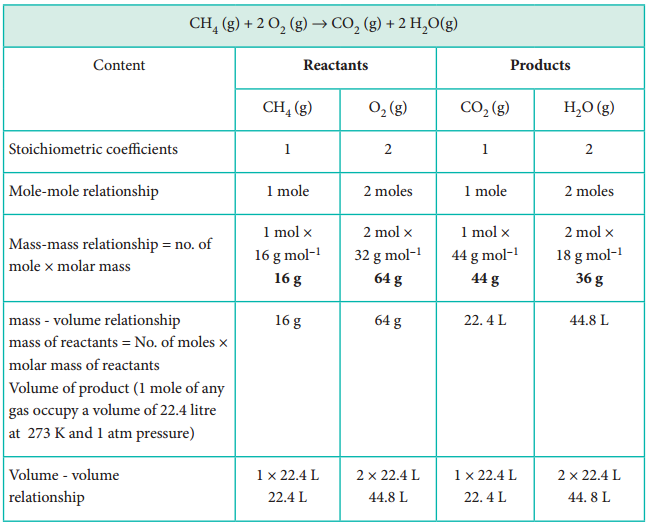Calculations Based on Stoichiometry

1. How many moles of hydrogen is required to produce 10 moles of ammonia?

The balanced stoichiometric equation for the formation of ammonia is

N2(g) + 3H2 (g) → 2 NH3 (g)

As per the stoichiometric equation, to produce 2 moles of ammonia, 3 moles of hydrogen are required

∴ to produce 10 moles of ammonia, 3 moles of hydrogen are required= 15 moles of hydrogen are required.

2. Calculate the amount of water produced by the combustion of 32 g of methane.

CH4(g) + 2O2(g) → CO2(g) + 2H2O(g)

As per the stoichiometric equation,

Combustion of 1 mole (16 g) CH4 produces 2 moles (2 × 18 = 36 g) of water.Combustion of 32 g CH4 produces3. How much volume of carbon dioxide is produced when 50 g of calcium carbonate is heated completely under standard conditions?

The balanced chemical equation is,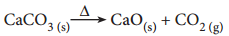As per the stoichiometric equation,

1 mole (100g) CaCO3 on heating produces 1 mole CO2At STP, 1 mole of CO2 occupies a volume of 22.7 litres

∴ At STP, 50 g of CaCO3 on heating produces,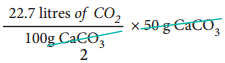= 11.35 litres of CO2

4. How much volume of chlorine is required to form 11.2 L of HCl at 273 K and 1 atm pressure?

The balanced equation for the formation of HCl is

H2(g) + Cl2(g) → 2 HCl (g)

As per the stoichiometric equation under given conditions,

To produce 2 moles of HCl, 1 mole ofchlorine gas is required

To produce 44.8 litres of HCl, 22.4 litres of chlorine gas are required.

∴ To produce 11.2 litres of HCl,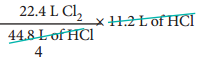= 5.6 litres of chlorine are required.

5. Calculate the percentage composition of the elements present in magnesium carbonate. How many kilogram of CO2 can be obtained by heating 1 kg of 90% pure magnesium carbonate.

The balanced chemical equation is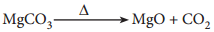Molar mass of MgCO3 is 84 g mol-1.

84 g MgCO3 contain 24 g of Magnesium.

∴ 100 g of MgCO3 contain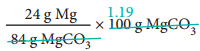= 28.57 g Mg.

i.e. percentage of magnesium
= 28.57 %.

84 g MgCO3 contain 12 g of carbon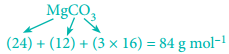∴ 100 g MgCO3 contain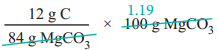= 14.29 g of carbon.

∴ Percentage of carbon
= 14.29 %.

84 g MgCO3 contain 48 g of oxygen
∴ 100 g MgCO3 contains∴ Percentage of oxygen
= 57.14 %.

As per the stoichiometric equation,

84 g of 100 % pure MgCO3 on heating gives 44 g of CO2.

∴ 1000 g of 90 % pure MgCO3 gives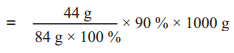= 471.43 g of CO2
= 0.471 kg of CO2

Limiting Reagents:

Earlier, we learnt that the stoichiometry concept is useful in predicting the amount of product formed in a given chemical reaction. If the reaction is carried out with stoichiometric quantities of reactants, then all the reactants will be converted into products.

On the other hand, when a reaction is carried out using non-stoichiometric quantities of the reactants, the product yield will be determined by the reactant that is completely consumed. It limits the further reaction from taking place and is called as the limiting reagent. The other reagents which are in excess are called the excess reagents.

Recall the analogy that we used in stoichiometry concept i.e. kesari preparation, As per the recipe requirement, 2 cups of sugar are needed for every cup of rava. Consider a situation where 8 cups of sugar and 3 cups of rava are available (all other ingredients are in excess), as per the cooking recipe, we require 3 cups of rava and 6 cups of sugar to prepare 18 cups of kesari.

Even though we have 2 more cups of sugar left, we cannot make any more quantity of Kesari as there is no rava available and hence rava limits the quantity of Kesari in this case. Extending this analogy for the  chemical reaction in which three moles of sulphur are allowed to react with twelve moles of fluorine to give sulphur hexafluoride.

The balanced equation for this reaction is, S + 3F2 → SF6

As per the stoichiometry,

1 mole of sulphur reacts with 3 moles of fluorine to form 1 mole of sulphur hexafluoride and therefore 3 moles of sulphur reacts with 9 moles of fluorine to form 3 moles of sulphur hexafluoride. In this case, all available sulphur gets consumed and therefore it limits the further reaction. Hence sulphur is the limiting reagent and fluorine is the excess reagent. The remaining three moles of fluorine are in excess and do not react.

Urea, a commonly used nitrogen based fertilizer, is prepared by the reaction between ammonia and carbon dioxide as follows.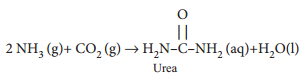In a process, 646 g of ammonia is allowed to react with 1.144 kg of CO2 to form urea.

1. If the entire quantity of all the reactants is not consumed in the reaction which is the limiting reagent?
2. Calculate the quantity of urea formed and unreacted quantity of the excess reagent.

The balanced equation is

2 NH3 + CO2

H2NCONH2 + H2O

1. The entire quantity of ammonia is consumed in the reaction. So ammonia is the limiting reagent. Some quantity of CO2 remains
unreacted, so CO2 is the excess reagent.

2. Quantity of urea formed

= number of moles of urea formed × molar mass of urea
= 19 moles × 60 g mol-1
= 1140 g
= 1.14 kg

Excess reagent leftover at the end of the reaction is carbon dioxide.

Amount of carbon dioxide leftver

= number of moles of CO2 left over × molar mass of CO2
= 7 moles × 44 g mol-1
= 308 g.